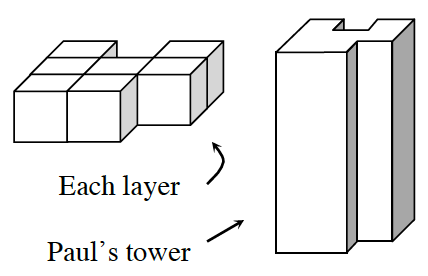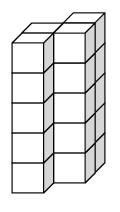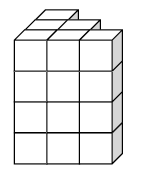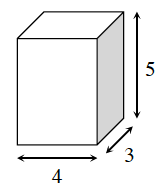### Home > INT2 > Chapter 10 > Lesson 10.1.1 > Problem10-6

10-6.Paul built a tower by stacking six identical layers of the shape at right on top of each other.

1. What is the volume of his tower? How can you tell without building the shape?

2. What is the surface area of his tower?

3. Paul’s tower is an example of a prism (refer to the Math Notes box in this lesson to learn more about prisms). For each of the prisms below, determine the volume and surface area.

(1)Remember the volume of a prism is (area of the base) · (height).

(2)The total surface area of a prism is the area of all of the external faces of the prism.

Try separating the calculations into: surface area of the base/top + surface area of the sides.

(3)Tower (1):
V $= 20$ units$^3$, SA $= 58$ units$^2$